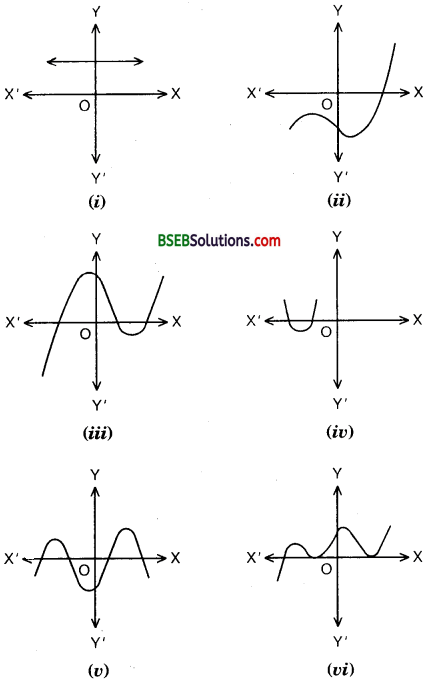# HSSlive: Plus One & Plus Two Notes & Solutions for Kerala State Board

## BSEB Class 10 Maths Chapter 2 Polynomials Ex 2.1 Textbook Solutions PDF: Download Bihar Board STD 10th Maths Chapter 2 Polynomials Ex 2.1 Book AnswersBSEB Class 10 Maths Chapter 2 Polynomials Ex 2.1 Textbook Solutions PDF: Download Bihar Board STD 10th Maths Chapter 2 Polynomials Ex 2.1 Book Answers

BSEB Class 10th Maths Chapter 2 Polynomials Ex 2.1 Textbooks Solutions and answers for students are now available in pdf format. Bihar Board Class 10th Maths Chapter 2 Polynomials Ex 2.1 Book answers and solutions are one of the most important study materials for any student. The Bihar Board Class 10th Maths Chapter 2 Polynomials Ex 2.1 books are published by the Bihar Board Publishers. These Bihar Board Class 10th Maths Chapter 2 Polynomials Ex 2.1 textbooks are prepared by a group of expert faculty members. Students can download these BSEB STD 10th Maths Chapter 2 Polynomials Ex 2.1 book solutions pdf online from this page.

## Bihar Board Class 10th Maths Chapter 2 Polynomials Ex 2.1 Books Solutions

 Board BSEB Materials Textbook Solutions/Guide Format DOC/PDF Class 10th Subject Maths Chapter 2 Polynomials Ex 2.1 Chapters All Provider Hsslive

## How to download Bihar Board Class 10th Maths Chapter 2 Polynomials Ex 2.1 Textbook Solutions Answers PDF Online?

2. Click on the Bihar Board Class 10th Maths Chapter 2 Polynomials Ex 2.1 Answers.
3. Look for your Bihar Board STD 10th Maths Chapter 2 Polynomials Ex 2.1 Textbooks PDF.
4. Now download or read the Bihar Board Class 10th Maths Chapter 2 Polynomials Ex 2.1 Textbook Solutions for PDF Free.

Find below the list of all BSEB Class 10th Maths Chapter 2 Polynomials Ex 2.1 Textbook Solutions for PDF’s for you to download and prepare for the upcoming exams:

## BSEB Bihar Board Class 10th Maths Solutions Chapter 2 Polynomials Ex 2.1

Question 1.
The graphs of y = p(x) are given in figures below for some polynomials p(x). Find the number of zeroes of p(x), in each case.Solution:

1. There are no zeros as the graph does not intersect the x-axis.
2. The number of zeroes is one as the graph intersects the x-axis at one point only.
3. The number of zeroes is three as the graph intersects the x-axis at three points.
4. The number of zeroes is two as the graph intersects the x-axis at two points.
5. The number of zeroes is four as the graph intersects the x-axis at four points.
6. The number of zeroes is three as the graph intersects the x-axis three points.

## Bihar Board Class 10th Maths Chapter 2 Polynomials Ex 2.1 Textbooks for Exam Preparations

Bihar Board Class 10th Maths Chapter 2 Polynomials Ex 2.1 Textbook Solutions can be of great help in your Bihar Board Class 10th Maths Chapter 2 Polynomials Ex 2.1 exam preparation. The BSEB STD 10th Maths Chapter 2 Polynomials Ex 2.1 Textbooks study material, used with the English medium textbooks, can help you complete the entire Class 10th Maths Chapter 2 Polynomials Ex 2.1 Books State Board syllabus with maximum efficiency.

## FAQs Regarding Bihar Board Class 10th Maths Chapter 2 Polynomials Ex 2.1 Textbook Solutions

#### Can we get a Bihar Board Book PDF for all Classes?

Yes you can get Bihar Board Text Book PDF for all classes using the links provided in the above article.

## Important Terms

Bihar Board Class 10th Maths Chapter 2 Polynomials Ex 2.1, BSEB Class 10th Maths Chapter 2 Polynomials Ex 2.1 Textbooks, Bihar Board Class 10th Maths Chapter 2 Polynomials Ex 2.1, Bihar Board Class 10th Maths Chapter 2 Polynomials Ex 2.1 Textbook solutions, BSEB Class 10th Maths Chapter 2 Polynomials Ex 2.1 Textbooks Solutions, Bihar Board STD 10th Maths Chapter 2 Polynomials Ex 2.1, BSEB STD 10th Maths Chapter 2 Polynomials Ex 2.1 Textbooks, Bihar Board STD 10th Maths Chapter 2 Polynomials Ex 2.1, Bihar Board STD 10th Maths Chapter 2 Polynomials Ex 2.1 Textbook solutions, BSEB STD 10th Maths Chapter 2 Polynomials Ex 2.1 Textbooks Solutions,
Share: